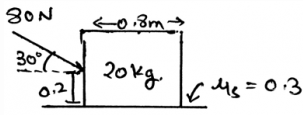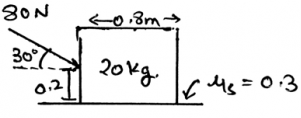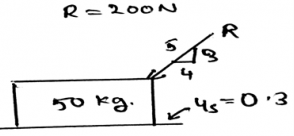# Test: Characteristics Of Dry Friction - 2

## 20 Questions MCQ Test Engineering Mechanics | Test: Characteristics Of Dry Friction - 2

Description
This mock test of Test: Characteristics Of Dry Friction - 2 for Mechanical Engineering helps you for every Mechanical Engineering entrance exam. This contains 20 Multiple Choice Questions for Mechanical Engineering Test: Characteristics Of Dry Friction - 2 (mcq) to study with solutions a complete question bank. The solved questions answers in this Test: Characteristics Of Dry Friction - 2 quiz give you a good mix of easy questions and tough questions. Mechanical Engineering students definitely take this Test: Characteristics Of Dry Friction - 2 exercise for a better result in the exam. You can find other Test: Characteristics Of Dry Friction - 2 extra questions, long questions & short questions for Mechanical Engineering on EduRev as well by searching above.
QUESTION: 1

### As we are using vector math to solve for the unknown forces in the free body diagrams, what is k.i?

Solution:

As the dot product of only the same Cartesian component is unity, i.e. i.i = 1 and j.j =1, rest all remaining dot product will give 0(i.j = 0 and j.k = 0). Cross product of the same plane vectors always give zero. This is generally done because the normal forces are perpendicular to the surfaces of action of the friction.

QUESTION: 2

### The three force system can also be in the equilibrium if:

Solution:

Even if the forces components cancels each other than the body is said to be in equilibrium. Also it should satisfy whether the moments caused by the forces are equal to zero or not. This means that we need to take components of the normal and frictional forces so as to make the desired calculations.

QUESTION: 3

### The normal forces and the forces of friction are collinear.

Solution:

They are not collinear. They are just acting perpendicular or parallel to the surface of action. Thus they are not even in the same plane. So as to make the calculations proper we need to see the same and then apply the equations.

QUESTION: 4

Determine the horizontal force acting in the given figure.Solution:

The net forces acting on the body is shown by the help of the resultant forces. There are two types, first the frictional and the second is the normal. This is because the resultant forces have the sum of all the forces which are acting on the direction which is same.

QUESTION: 5

Determine the vertical force acting in the given figure.Solution:

The net forces acting on the body is shown by the help of the resultant forces. There are two types, first the frictional and the second is the normal. This is because the resultant forces have the sum of all the forces which are acting on the direction which is same.

QUESTION: 6

The maximum value of the frictional force is called _________

Solution:

The friction is the phenomena that defines that there is a resistance which is present there between the two surfaces. The two surfaces are in contact and the friction applies at that surface only, resisting the motion of the surface. Thus the maximum values is called as limiting friction.

QUESTION: 7

When the body which is applied forces come in the stage of the limiting friction then the body is termed as to come in ___________ equilibrium.

Solution:

As the limiting friction is the maximum value of the frictional forces. Thus if more force is applied to the body then the body is going to move forward. Because the two surfaces are in contact and the friction applies at that surface only, resisting the motion of the surface. Thus the name unstable equilibrium.

QUESTION: 8

The frictional force is directly proportional to the ____________

Solution:

The frictional force is directly proportional to the vertical force that is being applied normal to the surface of the body. The force of friction is not dependent on the type of the surface. Thus the only thing the frictional force does depend is the normal force.

QUESTION: 9

The constant in the equation F = µN is called?

Solution:

The constant in the equation F = µN is known as the coefficient of static friction. This is the proportionality constant and is generally used to express the equation of the frictional force. The value of this constant is generally from 0 to infinite, depending on the surface.

QUESTION: 10

The coefficient of static friction does depend upon the surface on which the body is being slide.

Solution:

The constant in the equation F = µN is known as the coefficient of static friction. This is the proportionality constant and is generally used to express the equation of the frictional force. The value of this constant is generally from 0 to infinite, depending on the surface.

QUESTION: 11

The coefficient of friction is generally determined by ____________

Solution:

The coefficient of the friction is generally determined by the help of experiments. Many experiments are done on the body. Try and error methods are involved. And the final observations are being taken out. Then average of all the final answers resulted in the experiments is done.

QUESTION: 12

We have two types of coefficient of friction, one is coefficient of static friction and the other one is the coefficient of the kinetic friction.

Solution:

The constant in the equation F = µN is known as the coefficient of static friction. This is the proportionality constant and is generally used to express the equation of the frictional force. And in the same equation the constant is sometimes called coefficient of kinetic friction, when the limiting value of static friction is passed over.

QUESTION: 13

The angle of the inclination of wedge over which the block is sliding is determined by which of the following trigonometric function?

Solution:

The angle of the wedge over which the block is being slided is generally taken out by the help of the tangent inverse trigonometric function. It is the ratio of the frictional force to the normal force. This ratio is kept inside the inverse function.

QUESTION: 14

The coefficient of kinetic friction is ____________ than coefficient of static friction.

Solution:

The coefficient of kinetic friction is smaller than coefficient of static friction. The main thing about the kinetic one is that it is applied by the surface when the body is in motion. The static one is applied to the body when the body is static and is about to move.

QUESTION: 15

The kinetic friction is applied when the body is __________

Solution:

The kinetic friction is applied to the body by the surface when the body is moving. This means there is friction present and the coefficient of that friction is smaller than the static one. The main observation is that this is applied when the static friction attends its maximum value.

QUESTION: 16

The angle of the inclination of wedge over which the block is sliding and is experiencing the kinetic friction is determined by which of the following trigonometric function?

Solution:

The angle of the wedge over which the block is being slided is generally taken out by the help of the tangent inverse trigonometric function. Whether it may be the static or the kinetic friction, the ratio is the frictional force to the normal force. And this ratio is kept inside the inverse function.

QUESTION: 17

The value of coefficient of friction is taken at that moment when the block is at the verge of moving.

Solution:

The constant in the equation F = µN is known as the coefficient of static friction. This is the proportionality constant and is generally used to express the equation of the frictional force. And this coefficient is calculated by the help of experiments and is generally observed when the block is at the verge of moving.

QUESTION: 18

For determination of the equilibrium state in the free body diagram the basic way of getting the direction of the moment caused by the force is:

Solution:

The basic way of doing so is to use right hand rule and not the left hand rule. The direction of the moment axis is given by the thumb. The direction of the force is given by the fingers. As we place the fingers on the force and curl towards the rotational direction of the body about the axis.

QUESTION: 19

What is not the condition for the equilibrium in free body diagram for calculation of the normal forces?

Solution:

For the equilibrium in the three dimensional system of axis we have all the conditions true as, ∑Fx=0, ∑Fy=0 and ∑Fz=0. Also we have the summation of the forces equal to zero. Which is not a non-zero value.

QUESTION: 20

Which of the following is the force developed between the body and the surface.Solution:

The net forces acting on the body is shown by the help of the resultant forces. There are two types, first the frictional and the second is the normal. This is because the resultant forces have the sum of all the forces which are acting on the direction which is same.